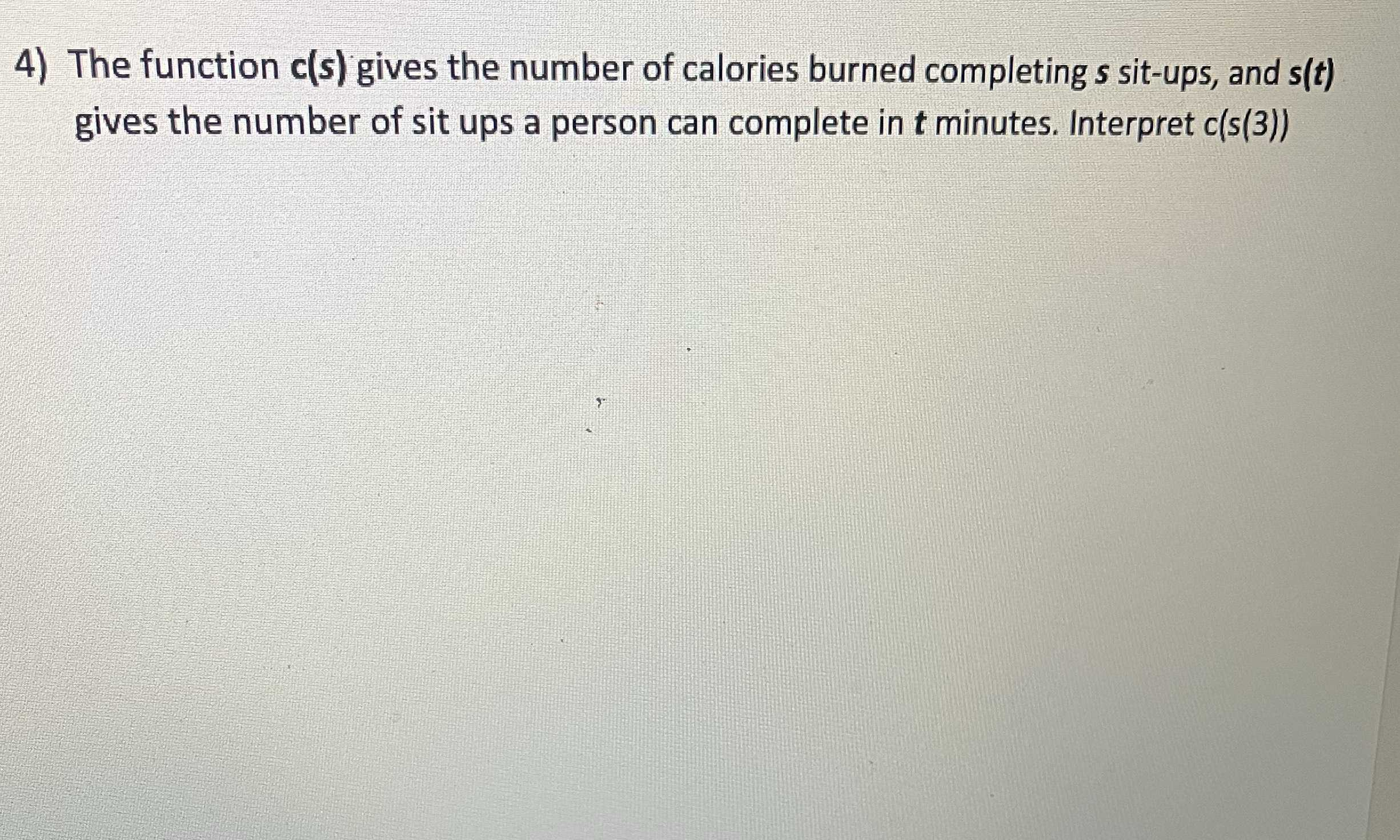### ¿Todavía tienes preguntas de matemáticas?

Pregunte a nuestros tutores expertos
Algebra
Pregunta4) The function $$c ( s )$$ gives the number of calories burned completing $$s$$ sit-ups, and $$s ( t )$$

gives the number of sit ups a person can complete in $$t$$ minutes. Interpret $$c ( s ( 3 ) )$$

$$the \ number \ of \ calories \ burned \ in \ 3 \ mins$$